# 数据结构：图的存储结构之邻接矩阵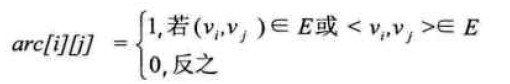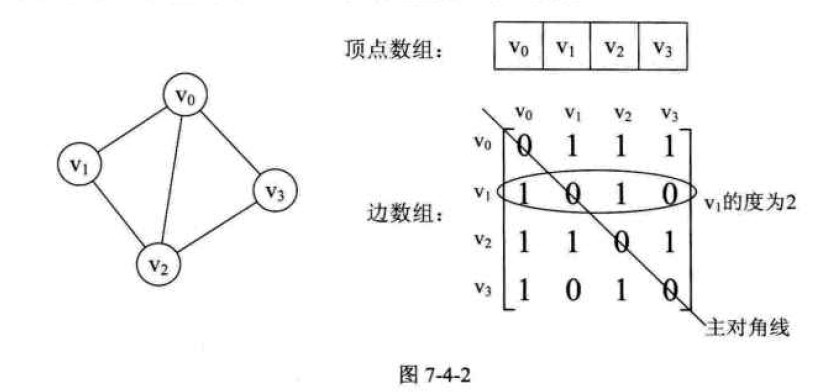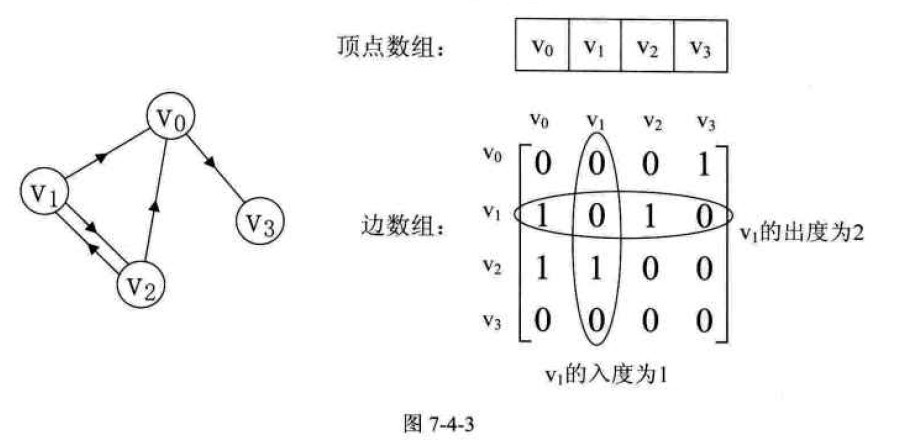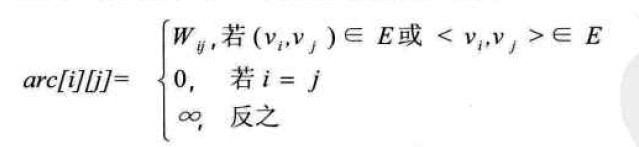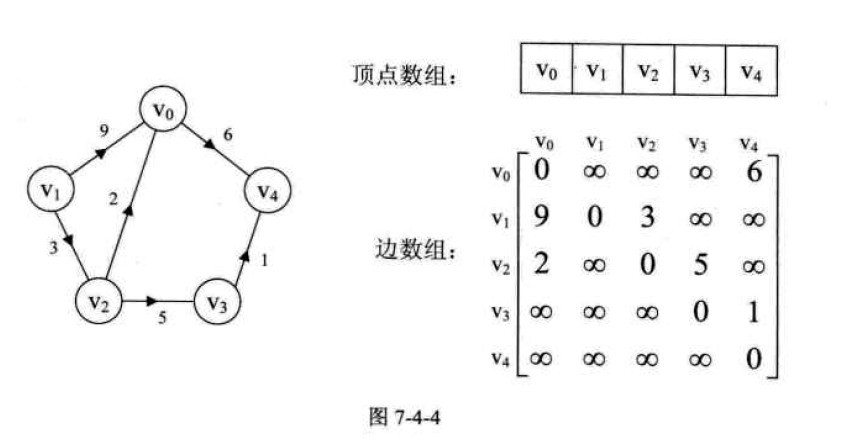C++ Code
 1 2 3 4 5 6 7 8 9 10 11 12 13 14 15 16 17 18 19 20 21 22 23 24 25 26 27 28 29 30 31 32 33 34 35 36 37 38 39 40 41 42 43 44 45 46 47 48 49 50 51 #includeusing namespace std;#define MAXVEX 100/* 最大顶点数，应由用户定义 */#define INFINITY  65535 /* 表示权值的无穷*/typedef int EdgeType;/* 边上的权值类型应由用户定义 */typedef char VertexType;/* 顶点类型应由用户定义  */typedef struct {     VertexType vexs[MAXVEX];/* 顶点表 */     EdgeType arc[MAXVEX][MAXVEX];/* 邻接矩阵，可看作边表 */     int numNodes, numEdges;/* 图中当前的顶点数和边数  */ } MGraph;/* 建立无向网图的邻接矩阵表示 */void CreateMGraph(MGraph *Gp) {     int i, j, k, w;     cout << "请输入顶点数和边数（空格分隔）：" << endl;     cin >> Gp->numNodes >> Gp->numEdges;     cout << "请输入顶点信息（空格分隔）：" << endl;     for (i = 0; i < Gp->numNodes; i++)         cin >> Gp->vexs[i];     for (i = 0; i < Gp->numNodes; i++)     {         for (j = 0; j < Gp->numNodes; j++)         {             if (i == j)                 Gp->arc[i][j] = 0;/* 顶点没有到自己的边*/             else                 Gp->arc[i][j] = INFINITY;/* 邻接矩阵初始化 */         }     }     for (k = 0; k < Gp->numEdges; k++)     {         cout << "请输入边（vi, vj)的上标i，下标j和权值w（空格分隔）:" << endl;         cin >> i >> j >> w;         Gp->arc[i][j] = w;         Gp->arc[j][i] = Gp->arc[i][j];/* 因为是无向图，矩阵对称 */     } }int main(void) {     MGraph MG;     CreateMGraph(&MG);     return 0; }

©️2019 CSDN 皮肤主题: 编程工作室 设计师: CSDN官方博客